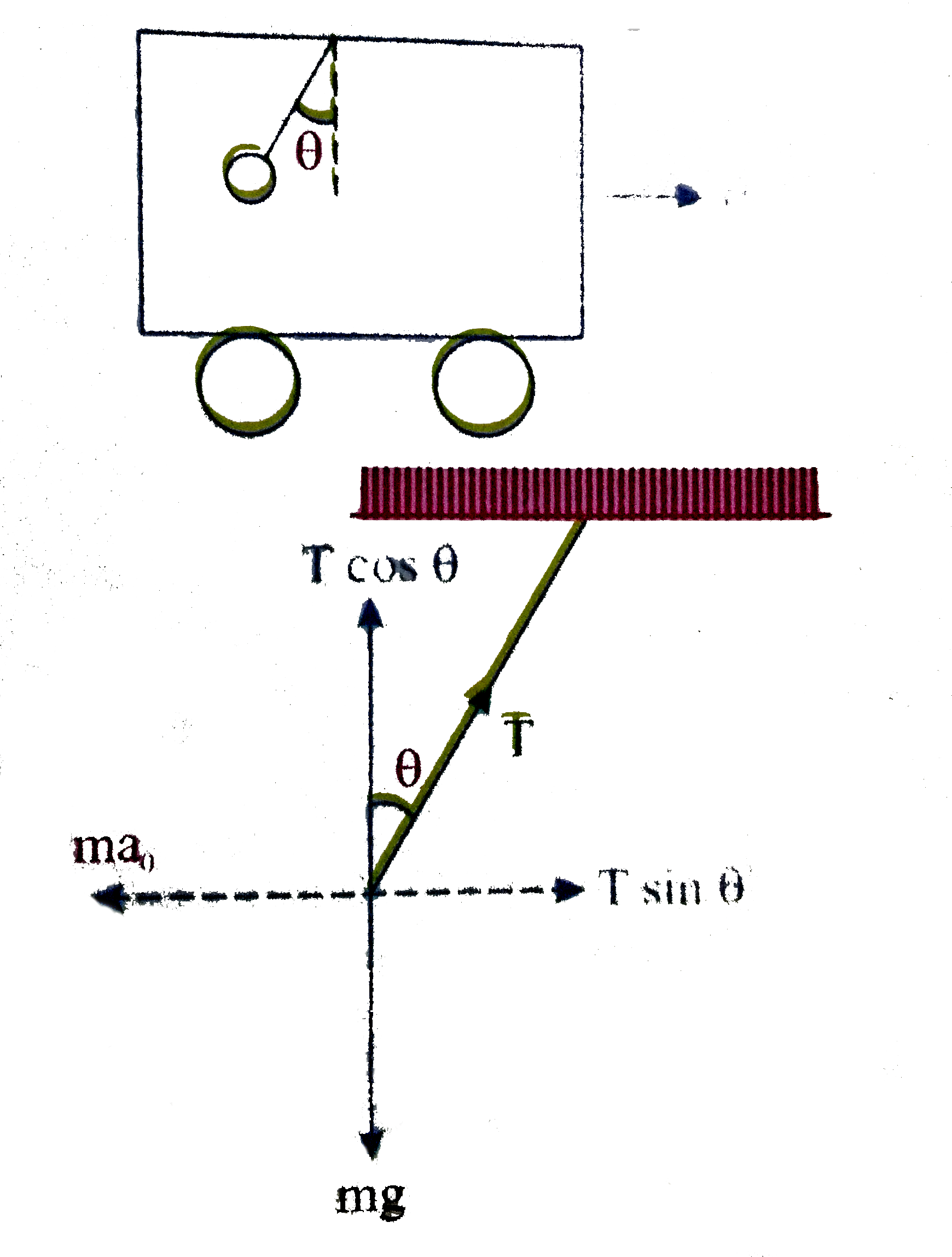# A pedulum is hanging from the ceiling of a car having an acceleration a_(0) with respect to the road Find the angle made by the string with vertical

30 views
in Physics
closed
A pedulum is hanging from the ceiling of a car having an acceleration a_(0) with respect to the road Find the angle made by the string with vertical at equilibrium Also find the tension in the string in this position.

by (78.8k points)
selected

T sin theta = ma_(0)….(i), T cos theta =mg….(ii)
dividing (i) & (ii) tan theta = (a_(0))/(g)
:. The string is making an angle theta = tan^(-1) ((a_(0))/(g)) with the vertical equilibrium
Squaring and adding (i) and (ii)
T^(2) sin^(2) theta +T^(2) cos^(2) theta =m^(2) (a_(0)^(2)+g^(2))
T =m sqrt(a_(0)^(2) +g^(2)) .